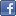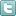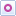EE Times-India > Sensors/MEMS

Sensors/MEMS# Utilising FETS for sensor applications (Part 1)

Posted: 09 Jul 2015Print Version

Keywords:field effect transistors  FETs  amplifier  accelerometers  transducers

There are a variety of field effect transistors (FETs) suitable for use in low noise amplifier applications for photo diodes, accelerometers, transducers, and other types of sensors. In particular, low noise JFETs exhibit low input gate currents that are desirable when working with high impedance devices at the input or with high value feedback resistors (e.g., ≥1MΩ). Operational amplifiers (op amps) with bipolar transistor input stages have much higher input noise currents than FETs.

In general, many op amps have a combination of higher noise and input capacitance when compared to some discrete FETs. For example, a typical FET input op amp may have input capacitances of about 20 pF, whereas many discrete FETs have input capacitances of less than 5 pF. Also, there are few low noise FET input op amps that have equivalent input noise voltages density of less than 4 nV/√Hz. However, there are a number of discrete FETs rated at ≤ 2 nv/√Hz in terms of equivalent input noise voltage density.

For those op amps that are rated as low noise, normally the input stages use bipolar transistors that generate much greater noise currents at the input terminals than FETs. These noise currents flowing into high impedances form added (random) noise voltages that are often much greater than the equivalent input noise.

One advantage of using discrete FETs is that an op amp that is not rated as low noise in terms of input current can be converted into an amplifier with low input current noise. For example, see the circuit shown in figure 1.Figure 1: Enhancing the noise performance of a bipolar input stage op amp.

In figure 1, current source Q2, JFETs J1A and J1B with load resistors R1A and R1B form a preamp to the input of U1 to provide better noise performance in terms of input bias current noise and equivalent input noise voltage. The collector current of Q2 is approximately 1V across R3 or about 4 mA. The drain currents of J1A and J1B are equal when Vin = 0, or about 2 mA each. With the load resistors set at 2KΩ, there are about 4V DC across each of these resistors. The typical transconductance, gm, for an LSK489 matched dual JFET is about 3 mS = 3 mmho at 2 mA drain current. Thus, the differential mode gain from the gates of J1A and J1B to the drains of J1A and J1B will be approximately 3 mS x 2KΩ = 6.

Note: 1 mho = 1 S = 1 amp/volt, and 1 mmho = 1 mS = 1 ma/volt.

NE5534 op amp comparison
Although an NE5534 op amp has about 4 nv per root Hertz in terms of equivalent noise voltage density, its input bias noise current in the order of 0.60 pA per root Hertz.

The DC gate current of a JFET will typically be less than 0.1 nA, and the input noise current will be:

2qIgB = noise current from the gate of the JFET

Ig = gate bias current

q = electron charge = 1.6 x 10-19C

B = bandwidth in Hertz. For a noise density calculation, the bandwidth is 1Hz. Thus, B = 1.

For 0.1 nA = Ig.

2qIgB = 0.00566 pA/√Hz = noise density current from the gate of the JFET.

In comparison to 0.60 pA/√Hz for the input noise density current for the NE5534, the JFET has about 100 times lower input noise current at 0.00566 pA/√Hz. The LSK489 has 1.8 nV/√Hz of noise voltage density per FET.

For J1A and J1B to be a dual matched JFET transistor such as the LSK489, the equivalent input noise voltage will be about 2.54 nV/√Hz, or about 3.925 dB lower noise than the 4 nV/√Hz rating of the NE5534.

Comment on "Utilising FETS for sensor applicatio..."

Top Ranked Articles

WebinarsVisit Asia Webinars to learn about the latest in technology and get practical design tips.

Search EE Times India
Services

Go to topConnect on FacebookFollow us on TwitterFollow us on Orkut
﻿

Back to Top